# Describing Off-Axis Conic Surfaces for Non-Axisymmetric Surface Generation

Two axes of motion are used by a conventional single point diamond turning (SPDT) lathe to describe a curve in space. The curve is formed between the centerline of the work piece to be machined and the cutting edge of a diamond tool. The rotation of the workpiece generates a 3D surface of revolution through the cutting action.

Due to the simplicity of these motions, the SPDT process has been limited to the generation of axisymmetric surfaces. The latest introduction of four axis SPDT has provided a technique for generating non-axisymmetric surfaces.

Further to the two axes of motion (X and Y) available on a SPDT lathe, two additional motions are used by four axis machining. The third motion is created by encoding the work holding spindle (φ axis) such that the machine tool controller can access the work piece’s angular position at the time of machining.

The fourth axis of motion, the z' axis, is an additional linear axis holding the SPDT tool. It has a limited range of motion but can enable extremely rapid positioning. A non-axisymmetric surface can be generated by coordinating the motion of the tool as a function of the work piece's angular position.

The machining of off-axis segments for large mosaic mirrors is one application of this technology. Conventionally for SPDT of these surfaces, the segment had to be positioned at the appropriate distance from the work holding spindle axis, and the surface was machined as part of a full parent surface of revolution. This method poses a restriction on the extent to which a segment can be off-axis from the parent axis, so that the limit is the maximum machine tool swing geometry.

This restriction is mainly on the distance the segment is off-axis, and not its physical size. Even small segments would need large machine tools in the case that they were very far off-axis. By deploying a four axis approach to the machining of these surfaces, the segments can be positioned on axis with the right off-axis surfaces generated by four axis non-axisymmetric machining.

This four-axis approach to non-axisymmetric surface generation ensures that the surface geometry of these segments can be described in the right manner.

This article covers the geometry of off-axis conic surfaces such that it is geometrically accurate and capable of the fast real-time solution required by four axis positioning at high rotational speeds.

Considerations that reduce the range of motion of the fourth axis are studied. To help explain these considerations, the geometries of the Keck Telescope primary mirror are used as examples.

## Off-Axis Surface Geometry

A sagitta equation in a coordinate system describes most aspheric off-axis surfaces in a coordinate surface referenced to the parent surface, such as: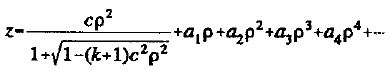(1)

This equation explains a conic surface of revolution modified by simple polynomial terms in a cylindrical coordinate system, with the origin of the coordinate system coincident with the vertex of the aspheric surface. The sagitta axis is the z axis, and it shows collinearity with the axis of rotation of the asphere.

In order to generate a non-axisymmetric off-axis section, a knowledge of the section geometry in a polar coordinate system centered on the off-axis surface is required. To attain this for the modified general aspheric surface, the solution of transcendental equations is neccessary.

It is possible to solve the case of the general conic of revolution in closed form, and that will be considered here. The benefit of a closed form description is that in a four-axis control system, the required motions can be coordinated in a "real time" environment.

Suitable representation of off-axis conic surfaces of revolution has been described in the literature for surfaces fabricated by deformation techniques. Approximations for typical non-axisymmetric surface fabrication, based on Zemike polynomials, have also been described. Thompson reported a precise description of off-axis paraboloid with restrictions on orientation. The derivation shown here for the general off-axis conic surface, tilted in the meridional plane at an arbitrary angle, follows the method of Thompson.

Thompson's method can be divided into four sections:

Firstly, the off axis geometry is described in the coordinate system of the parent conic surface, with the center of the off-axis segment along the x-axis. Next, it is converted to a second coordinate system, whose origin coincides with the center of the off-axis segment. It is further rotated in the meridional plane to a third coordinate system. Finally, it is translated from a Cartesian form to a cylindrical representation. Figure 1 shows the coordinate systems used.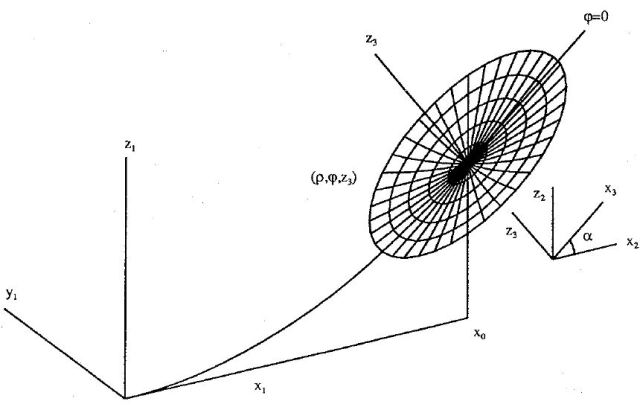Figure 1. Off-Axis Geometry

The derivation starts by describing the off-axis conic surface in the coordinate system of the parent surface of revolution. This initial description is given in equation 2, where r is the paraxial radius of curvature of the parent conicoid, and k is the conic constant.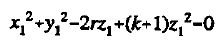(2)

By choosing a point (x0,0,z0) on the off-axis, it is possible to create a second coordinate system, using Equation 3 to translate the origin from the parent vertex to this new point. A new description of the centered off-axis surface can be written through direct substitution. After simplification, this gives Equation 4.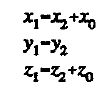(3)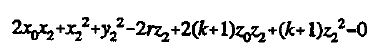(4)

By rotating the off-axis centered system through a meridional angle α, a third coordinate system is formed using Equation Set 5. After like terms are grouped and simplified, the description of the off-axis surface can be re-written in the form of a quadratic, equation 6, in the new sagitta direction z3.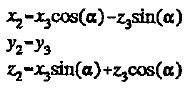(5)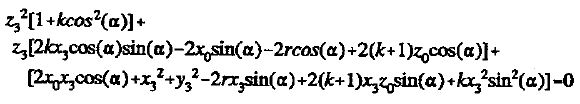(6)

The coordinate systems are finally transformed from Cartesian to cylindrical, using Equation set 7, offering the quadratic sagitta description, Equation 8, in the final orientation needed.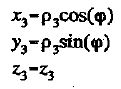(7)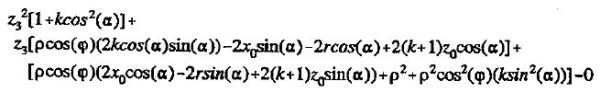(8)

After a considerable amount of simplification, the solution to this quadratic is given in the form of equation 9. Choosing this form allows the dependence of z3 on ρ and φ to be studied clearly.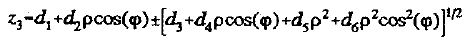(9)

Equations 10 to 15 define the new constants created.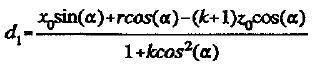(10)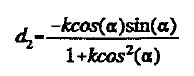(11)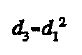(12)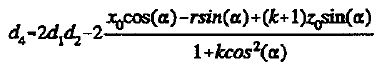(13)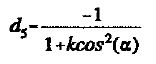(14)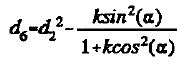(15)

There are far fewer restrictions on using this form. As the denominators share a common term, when its value is zero, the form fails. This can take place for hyperboloid segments tilted at certain angles, and for the unique case of a tilt free paraboloid. The choice of sign for the Equation 9 radical is determined by the sign of this denominator term. The form fails when the quantity below the radical is negative. This takes place when the value of ρ exceeds the range of the conicoid.

## Factors That Minimize Fourth Axis Motion

This description of the off-axis conic surface is suitable for manufacturing schemes that use only three (i.e. x, z, φ) axes of motion. It does not offer the separation of sagitta motions (i.e. z from z') necessary for a four axis machining scheme.

Due to the restricted motion range of the fourth axis, it is essential to divide the total sagitta motion, z, such that the fourth axis movement is minimized. This can be performed by choosing the right baseline of motion for the slow z-axis, and using the motion of the fast z'-axis to produce the required non-axisymmetric motion. Further to choosing an optimum baseline, choosing the right meridional tilt angle, α, can also reduce the fourth axis motion.

To show how the selection of baseline subtraction and tilt angle reduces fourth axis motions, the off-axis segments of the Keck Telescope primary mirror can be used an example. The popular tessellation of the Keck primary mirror is shown in Figure 2.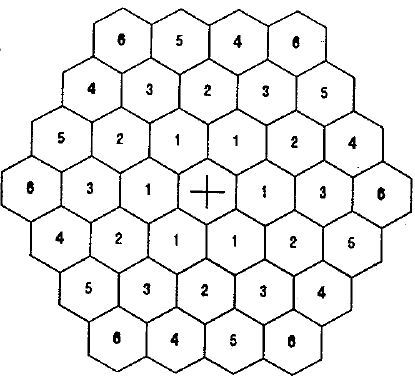Figure 2. Keck Primary Miror Tessellation

It is possible to group the 36 off-axis segments into six distinct surface shapes. Mirror segments of types 4 and 5 signify right and left hand versions of the same geometry. When observed along the axis of rotation of the hyperboloid primary, the outline of each projected section is hexagonal. Each hexagon’s edge length is 0.9 m.

The primary mirror for this Ritchey-Chretien telescope has a paraxial radius of curvature of 34.974 m and a conic constant of -1.003683. The sixth segment group geometry will be used for this example as it is the most non-axisymmetric.

A first estimate for the optimized meridional tilt angle is the surface slope at the off-axis center point (x0,0,z0), as viewed in the coordinate system of the original parent surface.

Though this estimate reduces the on-axisymmetric tool motion close to the center point, it fails to optimize the non-axisymmetric motion at any radial distance away from that point. When a tilt angle is selected that balances the figure in the meridional plane at the extreme radial positions, the overall non-axisymmetric geometry is reduced.

The variation between the original parent slope and the optimum slope for the Keck segments are within a few seconds of arc. The impact on fourth axis motion is a 20% reduction for the optimized tilt over the original slope. Figure 3 shows an anamorphically distorted view of the fourth axis motions for the Keck telescope’s outside segment with an optimized tilt angle and with the slope of the parent conic used. The baseline used for generating these images has the z-axis set to the value of z3 at φ=0 for each value of ρ.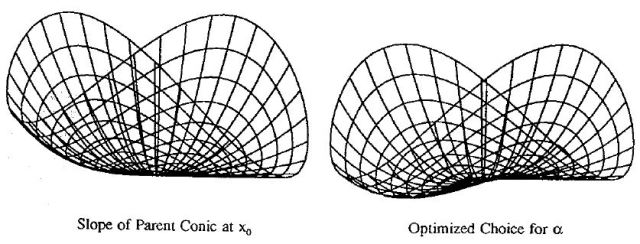Figure 3. Z' Fourth Axis Motion - Outside Keck Segment

(φ = 0° Baseline Subtracted)

Table 1 shows the tilt correction from the parent slope used to optimize the six geometries of the Keck primary mirror.

Table 1. Four Axis Parameters For The Keck Telescope Primary Mirror

row center position x0 tilt correction δα fourth axis motion z'
t1 1558.8 mm -3.10 arc sec 0.0235 mm
t2 2700.0 mm -5.31 arc sec 0.0701 mm
t3 3117.7 mm -6.10 arc sec 0.0932 mm
t4 4124.3 mm -7.93 arc sec 0.1617 mm
t5 4124.3 mm -7.93 arc sec 0.1617 mm
t6 4676.5 mm -8.88 arc sec 0.2067 mm

Choosing an optimum baseline is straightforward. For every radial position, the sagitta motion z3 has a maximum and a minimum value. By choosing the baseline to be the mean of these two values, the range of the fourth axis motion remains centered about a single position.

Two views of the outside keck segment are seen in Figure 4, with both the optimum tilt angle and optimum baseline subtracted. The wire frame model on the left is the surface as seen from the parent axis, and on the right from an orthogonal perspective. Table 1 shows values for optimized fourth axis motions for all six Keck geometries. It must be noted that for all these segments, the range of motion of the fourth axis required is very small compared to the dimensions of the segments themselves.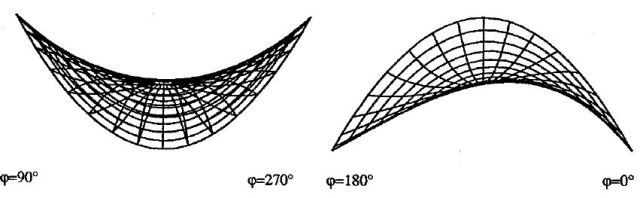Figure 4. Z' Fourth Axis Motion - Outside Keck Segment (Optimized Baseline Subtracted)

## Conclusion

This article offers the derivation of equations that describe the general off-axis conic surface. The equations derived are suited for the four axis generation of these surfaces in a non-axisymmetric manner. The factors reducing the range of motion have been studied.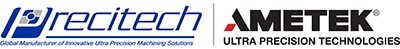This information has been sourced, reviewed and adapted from materials provided by Precitech.

## Citations

• APA

AMETEK Precitech. (2019, December 12). Describing Off-Axis Conic Surfaces for Non-Axisymmetric Surface Generation. AZoM. Retrieved on October 25, 2020 from https://www.azom.com/article.aspx?ArticleID=12289.

• MLA

AMETEK Precitech. "Describing Off-Axis Conic Surfaces for Non-Axisymmetric Surface Generation". AZoM. 25 October 2020. <https://www.azom.com/article.aspx?ArticleID=12289>.

• Chicago

AMETEK Precitech. "Describing Off-Axis Conic Surfaces for Non-Axisymmetric Surface Generation". AZoM. https://www.azom.com/article.aspx?ArticleID=12289. (accessed October 25, 2020).

• Harvard

AMETEK Precitech. 2019. Describing Off-Axis Conic Surfaces for Non-Axisymmetric Surface Generation. AZoM, viewed 25 October 2020, https://www.azom.com/article.aspx?ArticleID=12289.